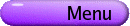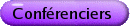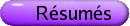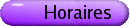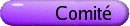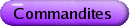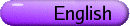Équations de Schrödinger non linéaires
Org: James Colliander et Robert Jerrard (Toronto)
[PDF]

IOAN BEJENARU, UCLA
Global solutions for Schroedinger Maps
[PDF]

We discuss the global theory for Schrödinger Maps in higher dimensions. For n ³ 3 we establish the global well-posedness for small initial data in the critical Besov space, while for n ³ 4 we establish the global well-posedness for small initial data in the critical Sobolev space.

PIETER BLUE, University of Toronto, Toronto, ON, M5S 2E4
Decay of waves on a warp product manifold with an unstable, closed geodesic surface
[PDF]

In this talk we compare the decay of solutions to the wave equation in R3 to those of the wave equation on a three-dimensional warp product space, (x,w) Î R ×S2 with metric dx2 +r(x)2 dw2. If the radii of spheres, r(x), has a unique minimum, then the sphere of minimal radius is a closed geodesic surface. Heuristically, this should impede the decay of waves, since waves follow the paths of geodesics. Indeed, Ralston has shown that initial data can be chosen for which an arbitrarily large percentage of the energy (H1 density) remains within a neighbourhood of the geodesic for arbitrary long periods of time. Thus, if decay estimates hold, there must be some loss of regularity, with higher derivatives used to control the decay of localised energy. The conformal charge is a weighted H1 norm defined by analogy to Rn, and its growth is controlled by the time integral of the energy near the geodesic surface. Using refinements of previous local decay arguments, the conformal charge can be shown to be bounded with an epsilon loss of regularity if the geodesic surface is unstable. This gives the same rate of decay for certain Lp norms as in Rn. This argument uses vector field techniques and can be extended to small data, nonlinear problems under additional assumptions on the growth of r(x). Since vector field techniques are analogous to commutator methods, we expect that similar methods will apply to the NLS on manifolds.

DANIELA DE SILVA, Johns Hopkins University
Low regularity solutions for a 2D quadratic non-linear Schrodinger equation
[PDF]

In this talk, we will discuss the initial value problem for the quadratic non-linear Schrödinger equation

 iut - Du = u2
where u : R2 ×R ® C. In a recent work in collaboration with I. Bejenaru, we proved that this problem is locally well-posed in Hs (R2) when s > -1. The critical exponent for this problem is sc = -1, and previous work of J. Colliander, J. Delort, C. Kenig and G. Staffilani, established local well-posedness for s > -3/4.

ROY GOODMAN, New Jersey Institute of Technology, Newark, NJ, USA
Fractal Structure in Solitary Wave Interactions
[PDF]

The following scenario has been seen in many non-integrable, dispersive, nonlinear PDE over the last 25 years: two solitary waves are propagated on a collision course. Above some critical velocity vc, they simply bounce off each other. Below vc they may be captured and merge into a single localized mass, or they may interact a finite number of times before escaping each other's embrace. Whether they are captured, and how many times the solitary waves interact before escape, depends on the initial velocity in a complicated manner, often remarked, though never shown, to be a fractal (a chaotic scattering process). This has been observed in coupled NLS, sine-Gordon, phi4, and others.

These PDE systems are commonly studied by (nonrigorously) deriving a reduced set of ODE that numerically reproduce this behavior. Using matched asymptotics and Melnikov integrals, we give asymptotic formulas for vc and for certain salient features of the fractal structure. We derive a discrete-time iterated map through which the entire structure can be unravelled.

Joint with Richard Haberman, Southern Methodist University.

MANOUSSOS GRILLAKIS, Maryland

NATASA PAVLOVIC, Princeton University, Department of Mathematics, Princeton, NJ 08544-1000, USA
Global well-posedness for the L2-critical NLS in higher dimensions
[PDF]

In this talk we will present a joint work with Daniela De Silva, Gigliola Staffilani and Nikolaos Tzirakis on global well-posedness for the L2 critical NLS in Rn with n ³ 3. Inspired by a recent paper of Fang and Grillakis, we combine the method of almost conservation laws with a local in time Morawetz estimate to improve global well-posedness results in higher dimensions.

ZOI RAPTI, University of Illinois at Urbana-Champaign
Modulational Instability for NLS equations with a periodic potential
[PDF]

This talk is about the stability properties of solutions to the NLS equation with a periodic potential which bifurcate from the Floquet-Bloch eigenstates of the linear problem in the small amplitude limit. We exploit the symmetries of the problem, in particular the fact that the monodromy matrix is a symplectic matrix. We find that the solutions corresponding to the band edges alternate stability, with the first band edge being modulationally unstable in the focusing case, the second band edge being modulationally unstable in the defocusing case, and so on.

LENYA RYZHIK, University of Chicago
Diffusion and flow mixing
[PDF]

I will describe some recent results on the interplay between diffusion and strong advection by an incompressible flow.

NIKOS TZIRAKIS, University of Toronto
Morawetz type inequalities and improved global well-posedness for the quintic NLS in 1d
[PDF]

Morawetz type estimates are monotonicity formulae that take advantage of the momentum conservation law of the nonlinear Schrödinger equation (NLS), and have been used extensively in obtaining global well-posedness and scattering results. By using an interaction Morawetz inequality for an "almost solution" of the NLS we prove a local-in-time Lt6 Lx6 bound. We use this bound along with the "I-method" to prove a new global well-posedness result for the quintic NLS in 1d.

This is joint work with D. De Silva, N. Pavlovic and G. Staffilani.

MONICA VISAN, Institute for Advanced Study, One Einstein Drive, Princeton, NJ 08540
The mass-critical NLS
[PDF]

We discuss recent progress on global well-posedness and scattering for the mass-critical NLS with initial data in L2.

The results presented are joint work with Terence Tao and Xiaoyi Zhang.

JIE XIAO, Memorial University, St. John's, NL, A1C 5S7
Old and New Morrey Spaces with Heat Kernel Bounds
[PDF]

In this talk I will address my work joint with D. Xuong and L. Yan. More precisely, given p Î [1,¥) and l Î (0,n), we discuss Morrey space Lp,l (Rn) of all locally integrable complex-valued functions f on Rn such that for every open Euclidean ball B Ì Rn with radius rB there are numbers C=C(f) (depending on f) and c=c(f,B) (relying upon f and B) satisfying

 rB-l óõ B |f(x)-c|p  dx £ C
and derive old and new, two essentially different cases arising from either choosing c = fB = |B|-1 òB f(y)  dy or replacing c by PtB(x) = òtB ptB (x,y) f(y)  dy-where tB is scaled to rB and pt (·,·) is the kernel of the infinitesimal generator L (taking the Schroedinger operator as a special one) of an analytic semigroup {e-tL}t ³ 0 on L2 (Rn). Consequently, we are led to simultaneously characterize the old and new Morrey spaces, but also to show that for a suitable operator L, the new Morrey space is equivalent to the old one.

Dispersion managed NLS and Strichartz inequality
[PDF]

Dispersion managed NLS is a model for optical pulse propagation in a fiber with piecewise constant dispersion. In a certain parameter regime, this equation possesses approximately periodic solitary waves called dispersion managed solitons. They turn out to be minimizers of an averaged variational principle. This variational principle is closely related to the Strichartz inequality. We will describe some interplay between these objects and in particular, we will present an easy proof of the classical version of the Strichartz inequality.

This is joint work with Dirk Hundertmark.

GANG ZHOU, University of Toronto
Perturbation Expansion and N-th Order Fermi Golden Rule of the Nonlinear Schrödinger Equations
[PDF]

In this presentation I will show the asymptotic stability of trapped solitions of generalized nonlinear Schrödinger equations with external potentials. We use Fermi Golden rule (FGR) to show the dynamics of the soliton which is close to Newton's equation plus a radiation term. We compute the expressions for the fourth and the sixth order Fermi Golden Rules (FGR) by perturbation expansion.